## Pricing Asian options in the Heston model

• The directory sites/default/files/tmp/drutex-25a95c3634899eff7a54f551ae0413ec-1 has been created.
• The directory sites/default/files/tmp/drutex-25a95c3634899eff7a54f551ae0413ec-2 has been created.
• The directory sites/default/files/tmp/drutex-25a95c3634899eff7a54f551ae0413ec-3 has been created.

Introduction

The following stochastic volatility model for the stock price dynamic in an incomplete market was introduced by Heston in 1993 . Under a Risk-Neutral probability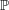, it writes: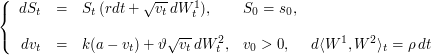whereand whereare such that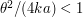. Here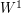and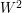are two standard Brownian motions under the probability measure. Consider the Asian Call option of maturity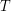and strikefor which there is no explicit formula: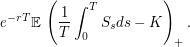As a first step, we projectonto, so thatwhereis a standard Brownian motion independent ofunder the probability measure. Then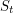writes

 TeX Embedding failed!

where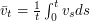.

Consider now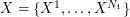and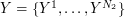two functional quantizers of the Brownian motion.

 TeX Embedding failed!

where tabs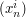andt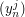are available here.

For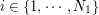and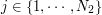, we numerically solve the following ordinary differential equations.

 TeX Embedding failed!

(Here, we used a Runge Kutta IV method.) The option price is now approximated byNumerical test

Here, we propose a method to compute the Asian call option price based on the functional quantization of the Brownian motion as described in article  (section 8).

Hereetare the sizes of optimal functional quantizers of the standard Brownian motion (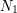"points", i.e. paths, foretfor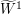). Parameterstands for the number of time-steps used to solve the ordinary differential equation written above. (We used a fourth order Runge Kutta scheme). Each grid couple yields a price approximation. The final result is a Romberg extrapolation between both prices, based on arate of convergence for the quadrature error.

The following program was developed with the C programming language, and interfaced with Ruby. You can contact the authors for the source code.

 Spot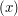Constant interest rateConstant dividend rate Initial varianceMean reversion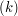Asymptotic variance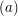Volatility of volatility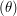Correlation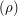Maturity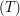Strike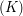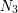This can take up to one minute of computation before displaying the results.

The site team would like to thank David Delavennat (CNRS ingeneer at LAMA-UMR 8050 Univ. MLV - Paris 12 from 2003 to 2007) for his advices on the Ruby interface.

### References

1. Steven L. Heston, The Review of Financial Studies , vol. 6, issue 2, pp. 327 - 343, 1993.
2. Gilles Pagès, and Jacques Printems, Monte Carlo Methods and Appl., vol. 11, no. 11, pp. 407-446, 2005.